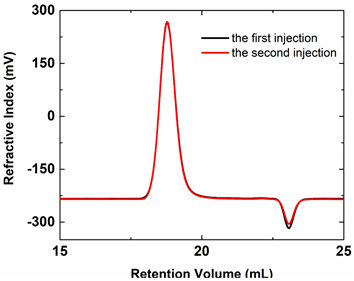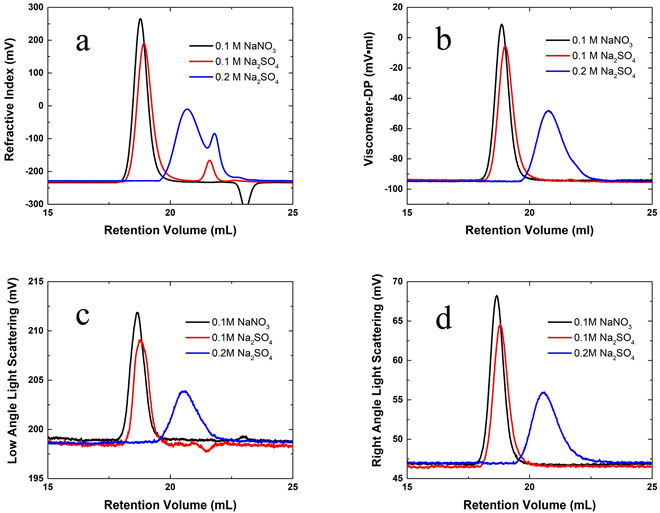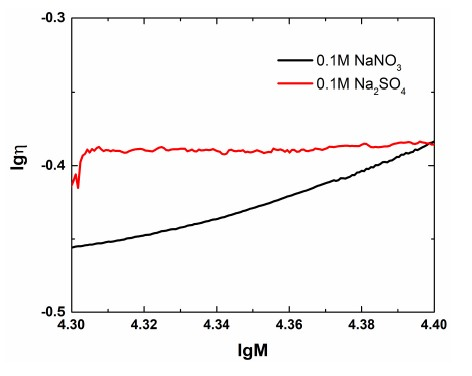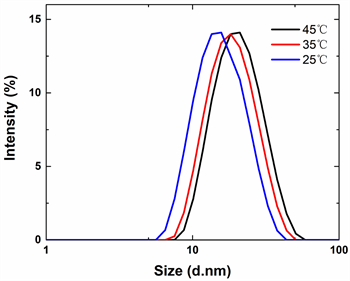﻿ 溶液中盐浓度对聚氧化乙烯构象的影响

溶液中盐浓度对聚氧化乙烯构象的影响Effect of Different Salt Solutions on the Conformation of Polyethylene Oxide

Abstract: The influence of salt concentration on the conformation of poly (ethylene oxide) in dilute solution was studied by using gel permeation chromatograph (TDA-GPC) coupled with differential light scattering and viscosity three detector coupled with dynamic light scattering (DLS) and polyoxy-ethylene standard sample (PEO-24K) as the research object. By comparing the physical parameters such as intrinsic viscosity (&eta;), hydrodynamic radius (Rh) and Mark-Houwink exponent (α) in different concentration of salt solution, the conformational changes of PEO were obtained: in lower concentration of salt solution, the typical random conformation of PEO was formed; with the increase of salt concentration, the molecular chain of PEO in solution gradually developed. The size of the coil decreases as the coil aggregates, shrinks and collapses, and the conformational change from random coil to densely packed collapse ball occurs. Meanwhile, the solution viscosity increases. This conformational change is mainly due to the reduction of solvation of PEO chains due to the competition between salt molecules in solution and water in PEO solution. The effect of temperature on the conformation of PEO has also been studied. When the temperature decreases, the molecular chain of PEO shrinks from random coils to collapsed spheres.

Abstract:

Abstract:

1. 引言

2. 实验部分

2.1. 试剂与仪器

2.2. 实验过程

2.2.1. TDA-GPC

2.2.2. DLSTable 1. Refractive index and viscosity of three solvents

3. 结果与讨论

TDA-GPCFigure 1. The RI curves of PEO dissolved 0.1 mol/L NaNO3 by repeated injections in by TDA-GPC

${A}_{\text{RI}}={K}_{\text{RI}}\cdot \left(\frac{\text{d}n}{\text{d}c}\right)\cdot {\int }^{\text{​}}c\left(V\right)\text{d}V$ (1)Figure 2. Different detector signal curves of PEO dissolved in three solutions by TDA-GPC, a: signal curve of the RI detector; b: signal curve of viscosity detector; c: the low angle signal curve of the light scattering detector; d: the right angle signal curve of the light scattering detectorTable 2. Peak area of different signals

${A}_{\text{LS}}={K}_{\text{LS}}\cdot {\left(\frac{\text{d}n}{\text{d}c}\right)}^{2}\cdot {K}_{W}\cdot c$ (2)

TDA-GPC对Rh的计算公式如下：

${R}_{h}=\sqrt{\frac{3M\cdot \left[\eta \right]}{10\text{π}\cdot {N}_{A}}}$ (3)

DLS是测试溶液中颗粒流体力学粒径的更方便快捷的方法。DLS通过检测颗粒的布朗运动速度，记录做布朗运动的颗粒造成的散射光波动随时间的变化，通过累积距法拟合得到颗粒的布朗运动速度，即扩散系数D。然后根据Stokes-Einstein方程  得到颗粒的粒径信息。计算公式如下：

$D=\frac{{k}_{B}T}{3\text{π}\eta d}$ (4)Table 3. Rh of PEO in three solvents by DLS and TDA-GPCTable 4. The calculation results of PEO in three different solvents by TDA-GPC

${\eta }_{sp}=\frac{4DP}{IP-2DP}$ (5)

$\left[\eta \right]=\frac{{\left[2\left({}_{sp}-\mathrm{ln}\left({}_{sp}+1\right)\right)\right]}^{0.5}}{c}$ (6)

$n\left[\eta \right]~\mathrm{ln}M$ 作图，得到样品的Mark-Houwink曲线(图3)，并根据方程：

$g\left[\eta \right]=\mathrm{lg}K+\alpha \cdot \mathrm{lg}M$ (7)Table 5. The particle size of PEO solved in 0.1 M NaNO3 at different temperatures by DLSFigure 3. Mark-Houwink curves of PEO dissolved in two solutions by TDA-GPCFigure 4. Particle size of PEO solved in 0.1 M NaNO3 at different temperatures by DLS

4. 结论

NOTES

*通讯作者。

 Luo, Y., Bai, C.K. and Jiang, X.D. (2008) Study of Solution Polymerization of Polyethylene Oxide. Fine Chemicals.

 赵梓良, 李琦, 薛彦虎, 等. 定量体积排除色谱测定高分子双水相系统的组成和分子量分布[J]. 高等学校化学学报, 2016, 37(1): 167-173.

 李文波, 薛锋, 程鎔时. 聚乙烯醇水溶液反复冷冻过程中的溶剂化效应[J]. 高分子学报, 2008, 1(12): 1198-1203.

 Werzer, O., Warr, G.G. and Atkin, R. (2011) Conformation of Poly(Ethylene Oxide) Dissolved in Ethylammonium Nitrate. Journal of Physical Chemistry B, 115, 648.
https://doi.org/10.1021/jp110216k

 王冉, 梅花, 任文坛, 等. PEO类聚合物基体的改性及其高性能电解质材料研究的新进展[J]. 材料导报, 2016, 30(11): 63-67.

 Guo, Q. and Chang, S. (2017)Tetra-Detector Size Exclusion Chromatography Characterization of Molecular and Solution Properties of Soluble Microbial Polysaccharides from an Anaerobic Membrane Bioreactor. Frontiers of Environmental Science & Engineering, 11, 16.
https://doi.org/10.1007/s11783-017-0922-x

 左矩. 激光散射原理及在高分子科学中的应用[M]. 郑州: 河南科学技术出版社, 1994.

 娄本浊, 王少清, 张华东, 等. 光纤式动态光散射系统综述[J]. 光学仪器, 2007, 29(1): 89-94.

 章宇斌, 齐崴, 苏荣欣, 等. 动态光散射分析不同物化条件下酪蛋白的聚集行为及其胶束尺寸[J]. 分析化学, 2007, 35(6): 809-813.

 刘俊杰, 国凯. 样品浓度对动态光散射法测量颗粒粒径的影响及分析[J]. 计量技术, 2014(8): 10-13.

 Zhao, C., Chao, Z. and Kang, H. (2017) Gelation of Na-Alginate Aqueous Solution: A Study of Sodium Ion Dynamics via NMR Relaxometry. Carbohydrate Polymers, 169, 206-212.
https://doi.org/10.1016/j.carbpol.2017.03.099

 郁彩红, 虞大红, 秦原, 等. NaCl在聚电解质溶液中活度系数的实验测定[J]. 化工学报, 2001, 52(8): 738-741.

 Harding, S.E., Abdelhameed, A.S. and Morris, G.A. (2010) Molecular Weight Distribution Evaluation of Polysaccharides and Glycoconjugates Using Analytical Ultracentrifugation. Macromolecular Bioscience, 10, 714-720.
https://doi.org/10.1002/mabi.201000072

 Fiebrig, I., Vårum, K.M. and Harding, S.E. (1997) Colloidal Gold and Colloidal Gold Labelled Wheat Germ Agglutinin as Molecular Probes for Identification in Mucin/Chitosan Complexes. Carbohydrate Polymers, 33, 91-99.
https://doi.org/10.1016/S0144-8617(97)00028-3

 Qian, G., Cui, S.W. and Wang, Q. (2009) Microstructure and Rheological Properties of Psyllium Polysaccharide Gel. Food Hydrocolloids, 23, 1542-1547.
https://doi.org/10.1016/j.foodhyd.2008.10.012

 Bauer, D.R. and Ullman, R. (1980) Contraction of Polystyrene Molecules in Dilute Solution below the θ Temperature. Macromolecules, 13, 392-396.
https://doi.org/10.1021/ma60074a036

Top